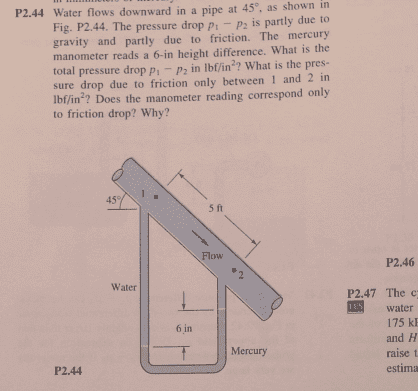# Fluid Mechanics: Manometry - Fluid through a pipe

• Feodalherren
In summary, the problem involves calculating the pressure drop between two points in a manometer using the hydrostatic equation. The solution involves determining the hydrostatic pressure drop in the manometer and subtracting it from the total pressure drop to find the frictional pressure drop. This is done by considering various points in the manometer and their respective elevations.

## Homework Statement## Homework Equations

Hydrostatic equation

## The Attempt at a Solution

Call length from 2 to the Hg "h".
Yhg = specific weight of Hg, from book = 846lbf/ft^3
Yw= water = 62.4 lbf / ft^3

Then

P2 = P1 + Yw(5sin45 + h + .5) - Yhg(.5) - Yw(h)

Then P2 - P1 = simplify and plug in numbers = 171.2 lbf/ft^2 = 1.19 PSI.

I get lost on the second part though. How am I supposed to know what is due to friction and which part the manometer reads? I'm assuming it ignores the weight of the fluid because it's the same in both places so it cancels out?

There is no flow through the manometer loop, so there is no frictional pressure drop in the manometer loop. Use the hydrostatic equation for the manometer loop to determine P2, given P1. Then get P1 - P2. This is the actual pressure drop between these two locations. If the main flow were hydrostatic, what would the pressure drop between P1 and P2 have been?

Chet

•Feodalherren
The solution claims that the manometer only reads the frictional drop so then if the main flow was static, then the pressure drop would have been 0...?

Feodalherren said:
The solution claims that the manometer only reads the frictional drop so then if the main flow was static, then the pressure drop would have been 0...?
If you recognize that the hydrostatic component of the pressure drop in the flow channel is also determined by the difference in elevation between points 1 and 2 in the manometer, then, if you apply what I said in my previous post, you will find that the frictional pressure drop is determined by the 6" difference in elevation of the mercury in the manometer times the difference in density between mercury and water. However, this result is far from obvious at first glance, and the approach I suggested helps you derive it.

Chet

•Feodalherren
I did the P2 - P1 part but I'm afraid I still don't get it. How exactly am I supposed to tell which term involves friction? I can find the term since you told me which one it is but I have no idea WHY that term is linked to friction. The way I see it, it could be any term.

Feodalherren said:
I did the P2 - P1 part but I'm afraid I still don't get it. How exactly am I supposed to tell which term involves friction? I can find the term since you told me which one it is but I have no idea WHY that term is linked to friction. The way I see it, it could be any term.
You are aware that the total pressure drop is the linear sum of the hydrostatic pressure drop and the frictional pressure drop, correct? The hydrostatic pressure drop is just determined by the vertical height difference between points 1 and 2.

Let's focus on the manometer. Let's label some points on the diagram. In the left column, label point A as the location at the same elevation as point 2 (the top of the right manometer tube). Label point B in the left column as the location at the same elevation with the top of the mercury in the right column. Label point C in the left column as the location at the top of the mercury in the left column. Label point D in the right column as the location opposite point C in the left column. Label point E in the right column as the top of the mercury in the right column. The total pressure difference between points 1 and 2 is the sum of the hydrostatic contribution and the frictional contribution within the flow channel. It is also equal to the purely hydrostatic contributions around the manometer from point 1 to point 2. The hydrostatic contribution for the flow channel is determined by the difference in elevation between points 1 and 2, irrespective of whether we are considering the flow channel or whether we are considering the manometer. So the hydrostatic pressure drop between point 1 and point A in the left manometer arm is exactly equal to the hydrostatic contribution to the pressure drop in the flow channel. Therefore, the frictional pressure drop in the flow channel must be equal to the pressure difference between point A in the left arm of the manometer and point 2 in the right arm of the manometer.

Within the manometer, the pressure difference between points A and B in the left arm exactly cancels the pressure difference between point E and point 2 in the right arm. The pressure difference between point C in the left are and point D in the right are must be zero (because they are both at the same elevation and there is only mercury between them). So, after all these considerations, the frictional pressure drop must be equal to the hydrostatic pressure difference for the mercury between points D and E in the right arm minus the hydrostatic pressure difference for the water between points C and B in the left arm.

I hope this makes sense.

Chet

•Feodalherren
That makes a ton of sense. Thank you very much!